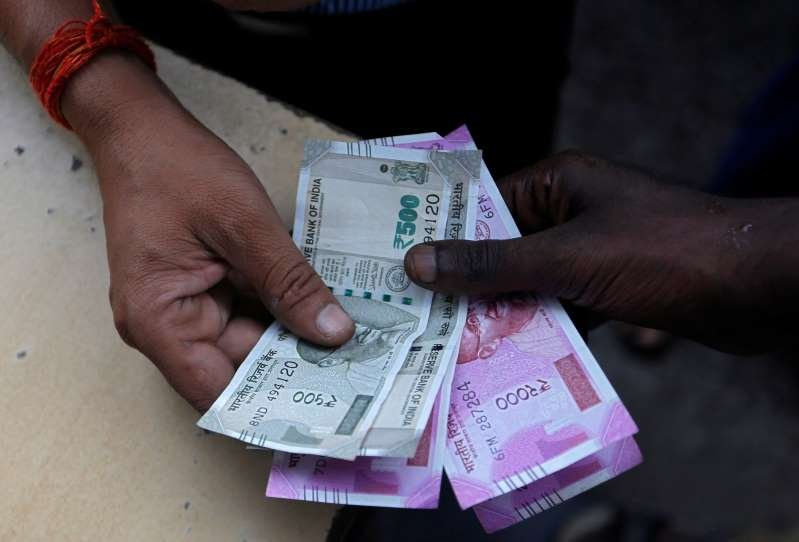in

# Here’s how many lakhs are needed in FD, MF, PPF, Provident FundGetting at least Rs 50,000 per month out of the investments made would be everyone’s desire. There are many investment avenues available to you to accumulate the required amount to enjoy the monthly return. Some of the popular investments options are fixed deposit (FD), mutual fund (MF), Public Provident Fund (PPF) and Employees’ Provident Fund (PF).

Out of the four options, FD is predominantly used for getting returns by investing a lump sum money, but the cumulative option may be used for accumulation also. MFs are also used for duel purpose, but equity MFs are predominantly used for accumulation of fund. PPF and PF, however, are used for accumulation purpose only.

As provident funds are meant for accumulation of retirement corpus, they are undoubtably long-term investment options. While the tenure of PPF is 15 years, PF is used for accumulating retirement corpus throughout working life of a private sector employee. Taking the long-term aspects, let’s assume accumulation period of 25 years.

Now, let’s see how much corpus would be needed to get Rs 50,000 monthly or Rs 6 lakh annually by investing the amount in FD. Assuming that the average current FD rate of 7 per cent per annum would remain constant, to get Rs 6 lakh annually, the lump sum amount to be invested is about Rs 85,71,500.

To accumulate Rs 85,71,500 in 25 years, annual investment needed at the beginning of every year under various investment options would be as follows.

FD: At an interest rate of 7 per cent per annum, Rs 1,26,654 are to be invested at the beginning of every year for 25 years to accumulate Rs 85,71,500.

MF: Assuming a long-term interest rate of 12 per cent per annum, about Rs 57,398 should be invested in equity MFs at the beginning of every year for 25 years to accumulate Rs 85,71,500.

PPF: Assuming that the current interest rate of 8 per cent per annum will remain unchanged, Rs 1,08,563 should to be invested at the beginning of every year for 25 years to accumulate Rs 85,71,500.

PF: Assuming that the current interest rate of 8.65 per cent per annum will remain unchanged, Rs 98,090 need to be invested at the beginning of every year for 25 years to accumulate Rs 85,71,500.